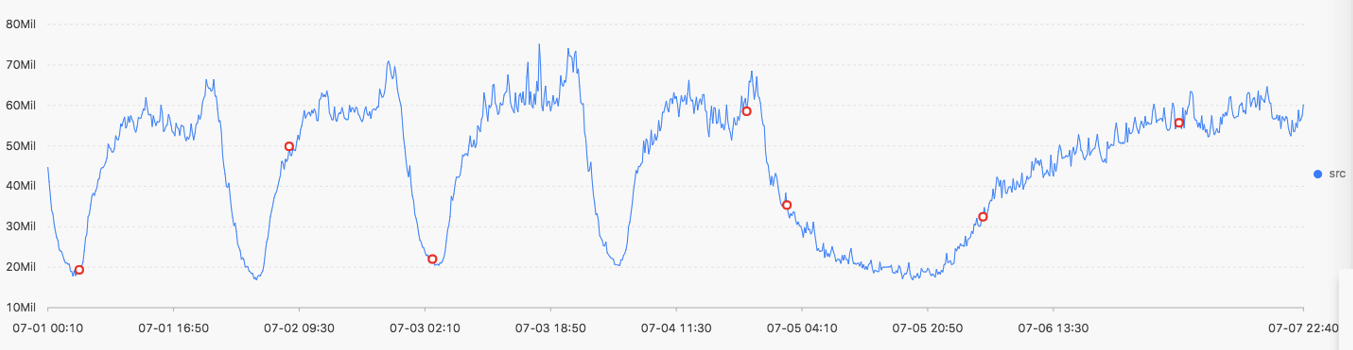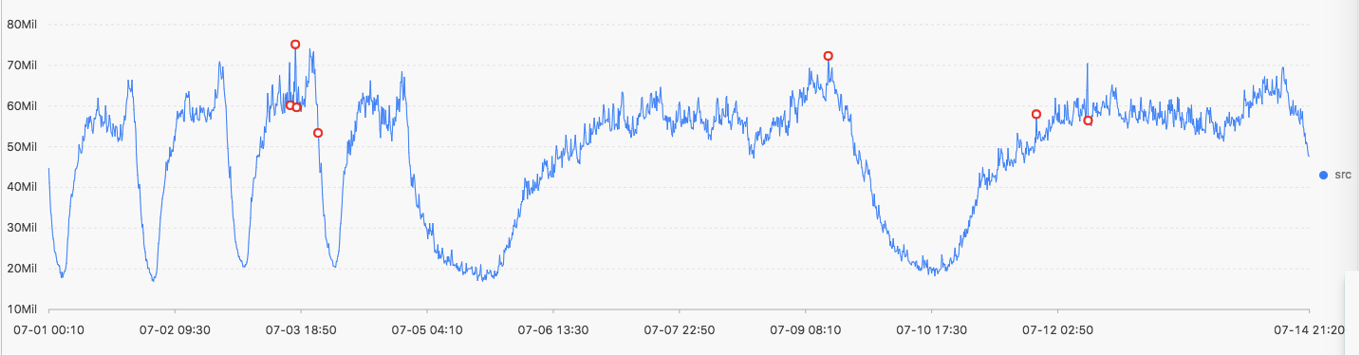The change point detection function detects change points in time series data.

The change point detection function supports two types of change points:
• Changes of statistical characteristics within a specified time period
• Obvious faulting in a sequence

Function list

Function Description
ts_cp_detect This function finds intervals with different statistical characteristics within a time series. The interval endpoints are change points.
ts_breakout_detect This function finds the time point when statistics steeply increase or decrease within a time series.

ts_cp_detect

Function format:
• If you are not sure about the window size, use the ts_cp_detect function in the following format. Then, the algorithm called by the function will use a window with a length of 10 to detect change points.

select ts_cp_detect(x, y, amplePeriod,sampleMethod)
• If you need to adjust the display effect of a service curve, use the ts_cp_detect function in the following format. Then, you can optimize the display effect by setting the minSize parameter.

select ts_cp_detect(x, y, minSize, samplePeriod, sampleMethod)
The following table describes the parameters.
Parameter Description Value
x Time column in ascending order Unixtime timestamp in seconds
y Numeric column corresponding to the data at a specified time point -
minSize Minimum length of consecutive intervals The minimum value is 3, and the maximum value cannot exceed 1/10 of the length of the current input data.
samplePeriod Period during which the current time series data is sampled Long type values ranging from 1 to 86399 seconds
sampleMethod Method for sampling the data in the sampling window Value range:
• avg: average value of the data in the window
• max: maximum value of the data in the window
• min: minimum value of the data in the window
• sum: sum of the data in the window
Example:
• Statement for query and analysis:
* | select ts_cp_detect(stamp, value, 3, 1, 'avg') from (select __time__ - __time__ % 10 as stamp, avg(v) as value from log GROUP BY stamp order by stamp)
• Result:The following table describes the display items.
Display item Description
Horizontal axis unixtime Data timestamp in seconds, for example, 1537071480
Longitudinal axis src Data before filtering, for example, 1956092.7647745228
prob Probability that a point is a change point. Its value ranges from 0 to 1.

ts_breakout_detect

Function format:
select ts_breakout_detect(x, y, winSize, samplePeriod, sampleMethod)
The following table describes the parameters.
Parameter Description Value
x Time column in ascending order Unixtime timestamp in seconds
y Numeric column corresponding to the data at a specified time point -
winSize Minimum length of consecutive intervals The minimum value is 3, and the maximum value cannot exceed 1/10 of the length of the current input data.
samplePeriod Period during which the current time series data is sampled Long type values ranging from 1 to 86399 seconds
sampleMethod Method for sampling the data in the sampling window Value range:
• avg: average value of the data in the window
• max: maximum value of the data in the window
• min: minimum value of the data in the window
• sum: sum of the data in the window
Example:
• Statement for query and analysis:
* | select ts_breakout_detect(stamp, value, 3, 1, 'avg') from (select __time__ - __time__ % 10 as stamp, avg(v) as value from log GROUP BY stamp order by stamp)
• Result:The following table describes the display items.
Display item Description
Horizontal axis unixtime Data timestamp in seconds, for example, 1537071480
Longitudinal axis src Data before filtering, for example, 1956092.7647745228
prob Probability that a point is a change point. Its value ranges from 0 to 1.